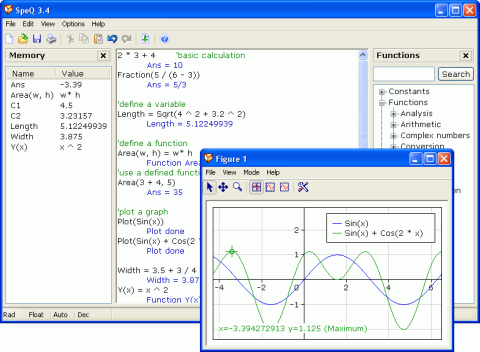## What is it

SpeQ is a small, extensive mathematics program with a simple, intuitive interface. All calculations are entered in a sheet. In there you can freely add, edit and execute calculations. SpeQ supports all common functions, constants, and units. Furthermore, you can define custom variables and functions, and plot graphs of your functions.

## Handy!

SpeQ is written to be an advanced but very easy to use math program. It is small and fast and runs on every Windows computer, also from an USB-key or floppy disk. SpeQ is useful for simple, brief calculations as well as working out sheets with extensive variable definitions, functions and complex calculations.

SpeQ is usable for engineers, students and pupils or simply as replacement of the Windows Calculator.## Features

SpeQ has the following features.

• Expression calculations with arithmetic, conditional, logical and bitwise operators
• Around 100 built-in mathematical, physical and conditional constants
• Around 60 built-in functions for Analysis, Arithmetic, Complex numbers, Hyperbolic, Integers, Logical, Probability, Numeral systems, Statistics, Trigonometry
• Define your own variables and functions for use in calculations
• Plot fully adaptable graphs, trace the plotted functions, save your graphs
• Extensive support for units
• Decimal, binary, hexadecimal and octal numeral systems
• Calculations with lists
• Complex number calculations
• Colored workarea
• Orderly overview of all available functions and constants in the Functionstree
• A list with all defined variables and functions in the Memorylist
• Context-sensitive help and a detailed users guide
More feature details...

• Enter mathematical expressions in a sheet
• Operators:
• Arithmetic: + - * / % ^ 2 3
• Conditional: =, <>, <, >, <=, >=
• Logical: And, Or, Xor
• Bitwise: &, |, ||
• Constants. Around 100 built-in constants on the area of
• Mathematics: General, Number Theory, Chaos Theory, Combinatorics, Analysis
• Physics: Universal, Electromagnetic, Atomic and Nuclear, Physico-Chemical
• Conditions
• Colors
• Functions:
• Analysis: fnInt, fnDiff, fnSolve
• Arithmetic: Abs, Exp, Ln, Log, Log10, Sqrt, Sign
• Complex numbers: Re, Im, Abs, Arg, Conj
• Hyperbolic: Cosh, Sinh, Tanh, ACosh, ASinh, ATanh, Sech, Csch, Coth
• Integers: Round, Floor, Ceil, Fix
• Logical: IIf, Not, Repeat, Iterate
• Probability: Factorial, nCr, nPr, !, Rand, RandInt
• Statistics: Avg, Min, Mean, Max, Prod, Sum, Std, Sort, Var
• Representation: Bin, Dec, Fraction, Hex, Oct
• Trigonometry: Cos, Sin, Tan, ACos, ASin, ATan, Atan2, Sec, Csc, Cot
• Plot graphs
• User-friendly trace, move and zoom functionality
• Analyze intersections, minima and maxima
• Adjust all figure properties in the Propertywindow or in the Workarea
• Available functions: Plot, PlotLogX, PlotLogY, PlotLogLog, RGB, Close, CloseAll
• Units. Around 130 built-in units on the area of
• Angles
• Mechanics: time, mass, length, area, volume
• Electricity
• Temperature
• Molecular
• Light intensity
• System functions and variables: Angles, Bytes, Decimals, SciNotation, Representation, Clear, ClearAll
• Define your own variables for use in calculations
• Define your own functions, using one or more variables
• Decimal, binary, hexadecimal and octal representation
• Calculations with lists
• Complex number calculations
• Colored workarea
• Orderly overview of all available functions and constants in the Functionstree
• A list with all defined variables and functions in the Memorylist
• Context-sensitive help and a detailed users guide

## System Requirements

SpeQ has very low system requirements. It runs on practically every Windows computer.

• Microsoft Windows 95, 98, ME, 2000, NT, XP, Vista, or 7
• Pentium 166 MHz or better processor
• 32 MB of RAM
• 1 MB free disk space